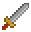# Vector

## Vectors

Excalibur uses the Vector structure to represent points. The Vector class has many different static methods available for doing vector math as well as instance methods to combine vectors together in different ways.

### Creating vectors

To quickly create a vector, use the vec global:

``````import { vec } from 'excalibur'

const point = vec(0, 10)``````

Alternatively, you may see examples of using the more verbose `new Vector(x, y)` format:

``````import { Vector } from 'excalibur'

const point = new Vector(0, 10)``````

To set the value of an existing vector, use Vector.setTo:

``````import { vec } from 'excalibur'

const point = vec(0, 10).setTo(10, 10)``````

There are some built-in vector constants you can use:

### Cloning vectors

Vectors are objects, so mutating them will change the state for all references. Use the Vector.clone method to clone a vector to mutate it:

``````import { vec } from 'excalibur'

const point = vec(0, 10)
const samePoint = point.setTo(8, 8)
const anotherPoint = point.clone().setTo(50, 50)

console.log(point.toString()) // "(8, 8)"
console.log(samePoint.toString()) // "(8, 8)"
console.log(anotherPoint.toString()) // "(50, 50)"``````

Notice how both `point` and `samePoint` share the same vector reference, so using `setTo` mutates the vector. Use `clone` to ensure you are not changing vectors unexpectedly!

##### Get Involved